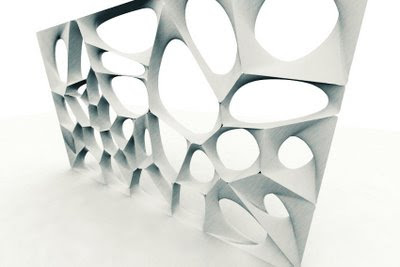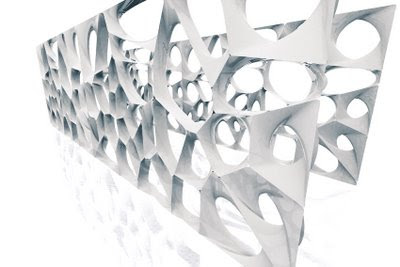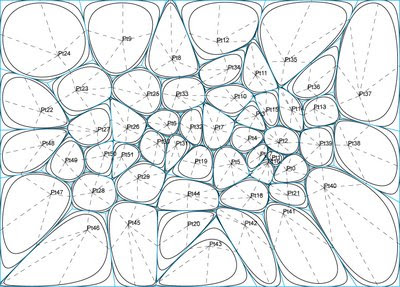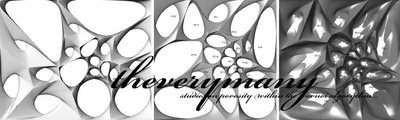## Monday, August 07, 2006

### Rh4_060807_VORNOIIn mathematics, a Voronoi diagram, named after Georgy Voronoi, also called a Voronoi tessellation, a Voronoi decomposition, or a Dirichlet tessellation (after Lejeune Dirichlet), is special kind of decomposition of a metric space determined by distances to a specified discrete set of objects in the space, e.g., by a discrete set of points.DEFINITION
For any (topologically) discrete set S of points in Euclidean space and for almost any point x, there is one point of S closest to x. The word "almost" is occasioned by the fact that a point x may be equally close to two or more points of S.

If S contains only two points, a and b, then the set of all points equidistant from a and b is a hyperplane — an affine subspace of codimension 1. That hyperplane is the boundary between the set of all points closer to a than to b, and the set of all points closer to b than to a. It is the perpendicular bisector of the line segment from a and b.

In general, the set of all points closer to a point c of S than to any other point of S is the interior of a (in some cases unbounded) convex polytope called the Dirichlet domain or Voronoi cell for c. The set of such polytopes tessellates the whole space, and is the Voronoi tessellation corresponding to the set S. If the dimension of the space is only 2, then it is easy to draw pictures of Voronoi tessellations, and in that case they are sometimes called Voronoi diagrams.

The dual for a Voronoi tessellation is the Delaunay triangulation for the same set of points S.GENERALIZATIONS
Voronoi cells can be defined for metrics other than Euclidean. However in these cases the Voronoi tessellation is not guaranteed to exist (or to be a "true" tessellation), since the equidistant locus for two points may fail to be subspace of codimension 1, even in the 2-dimensional case.

Voronoi cells can also be defined by measuring distances to areas rather than to points. These types of Voronoi cells are used in image segmentation, optical character recognition and other computational applications. In materials science, polycrystalline microstructures in metallic alloys are commonly represented using Voronoi tessellations.
ie WIKIPEDIAVORNOI CODE (from challenge last week at the office + Rassul...)
The Voronoi core has been developped by David Rutten (reconstructivism.net)

Function VoronoiPolygon(index, datSet, BBox)
' this function creates a voronoi cell for agiven point in a set of points
' should probably be optimized so that it only tests points near the samplepoint
VoronoiPolygon = Null

Dim midPt, arrPt, vecDir(1)
Dim ptS(2), ptE(2)
Dim ChordLength, Border
Dim brdLines(), i, N
ReDim brdLines(UBound(datSet)-1)
ChordLength = Rhino.Distance(BBox(0), BBox(2))

arrPt = datSet(index)
N = 0

For i = 0 To UBound(datSet)
If i <> index Then
midPt = Array((datSet(i)(0) + datSet(index)(0))/2, _
(datSet(i)(1) + datSet(index)(1))/2, _
0)
vecDir(0) = -(datSet(i)(1)-datSet(index)(1))
vecDir(1) = datSet(i)(0)-datSet(index)(0)
vecDir(0) = vecDir(0)/Rhino.Distance(datSet(i), datSet(index))*ChordLength
vecDir(1) = vecDir(1)/Rhino.Distance(datSet(i), datSet(index))*ChordLength
ptS(0) = midPt(0)+vecDir(0)
ptS(1) = midPt(1)+vecDir(1)
ptS(2) = 0
ptE(0) = midPt(0)-vecDir(0)
ptE(1) = midPt(1)-vecDir(1)
ptE(2) = 0
N = N+1
End If

Next

Border = Rhino.AddPolyline(Array(Array(BBox(0)(0)-10, BBox(0)(1)-10, 0), _
Array(BBox(1)(0)+10, BBox(1)(1)-10, 0), _
Array(BBox(2)(0)+10, BBox(2)(1)+10, 0), _
Array(BBox(3)(0)-10, BBox(3)(1)+10, 0), _
Array(BBox(0)(0)-10, BBox(0)(1)-10, 0)))
Rhino.UnselectAllObjects
Rhino.SelectObjects brdLines
Rhino.SelectObject Border
Rhino.Command "-_CurveBoolean _DeleteInput=No _CombineRegions=No " & _
Rhino.Pt2Str(Array(datSet(index)(0),datSet(index)(1),datSet(index)(2))) & _
" _Enter", vbFalse
' delete lines
Rhino.UnselectAllObjects
Rhino.SelectObjects brdLines
Rhino.Command "-_Delete"

VoronoiPolygon = vbTrue
End FunctionLabels: